# Texas Go Math Grade 5 Lesson 13.7 Answer Key Customary and Metric Conversions

Refer to our Texas Go Math Grade 5 Answer Key Pdf to score good marks in the exams. Test yourself by practicing the problems from Texas Go Math Grade 5 Lesson 13.7 Answer Key Customary and Metric Conversions.

## Texas Go Math Grade 5 Lesson 13.7 Answer Key Customary and Metric Conversions

Essential Question

How can you use the strategy to make a table to help you solve problems about customary and metric conversions?
Conversion factors are used in solving problems in which a certain measurement must be expressed with different units. When a given measurement is multiplied by an appropriate conversion factor, the numerical value changes, but the actual size of the quantity measured remains the same.

Unlock the Problem
Aaron is making fruit punch for a family reunion. He needs to make 120 cups of punch. If he wants to store the fruit punch in gallon containers, how many gallon containers will Aaron need?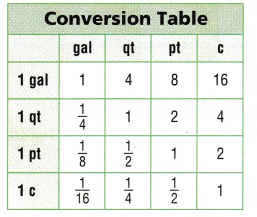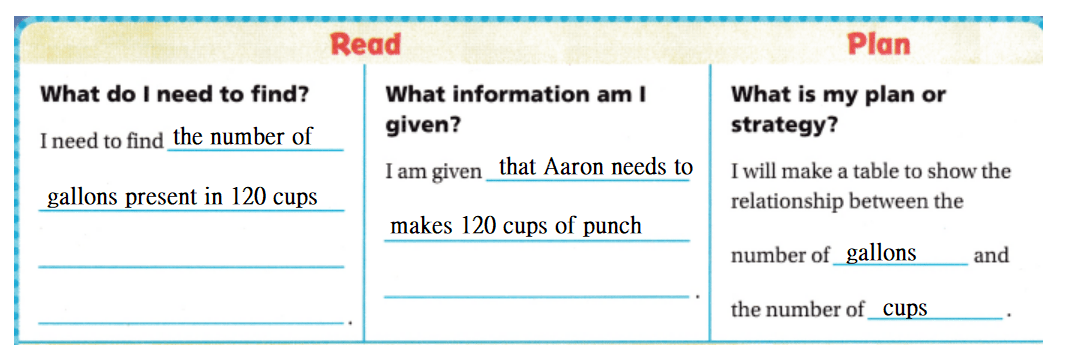Solve

There are 16 cups in 1 gallon. So, each cup is $$\frac{1}{16}$$ of a gallon.
Complete the table below.So, Aaron needs $$\frac{15}{2}$$ gallon containers to store the punch.

• Will all of the gallon containers Aaron uses be filled to capacity? Explain.
From the above problem,
We can observe that
Aaron needs $$\frac{15}{2}$$ gallon containers to store the punch
Now,
$$\frac{15}{2}$$ = 7.5
So,
All  of the 7-gallon containers will be filled and the 8th container will be half-filled
Hence, from the above.
We can conclude that
All the gallon containers of Aaron used are not filled to the capacity

Try Another Problem

Sharon is working on a project for art class. She needs to cut strips of wood that are each 1 decimeter long to complete the project. If Sharon has 7 strips of wood that are each 1 meter long, how many 1-decimeter strips can she cut?So,
Sharon can cut 70 1-decimeter lengths to complete her project.

• What relationship did the table shows that you made?
The given table is:Now,
From the given table,
We can observe that
The given table shows the relationship between the metric lengths and the customary lengths
Hence, from the above,
We can conclude that
The given table shows the relationship between the metric lengths and the customary lengths

Math Talk
Mathematical Processes
Explain how you could use another strategy to solve this problem.
The other strategy to solve the above problem is:
Step 1:
Write the given information
Step 2:
Formulate a strategy to solve the required information
Step 3:
Convert the metric units into customary units
Step 4:
Find the required quantity by using the given information

Share and Show

Question 1.
Edgardo has a drink cooler that holds 10 gallons of water. He is filling the cooler with a 1-quart container. How many times will he have to fill the quart container?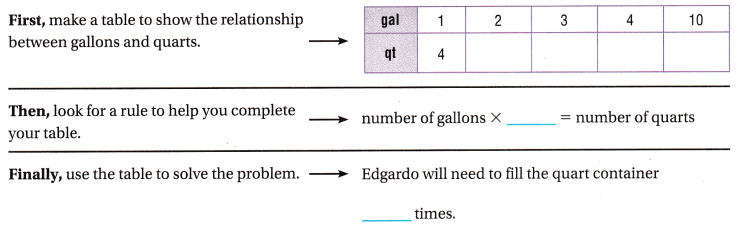It is given that
Edgardo has a drink cooler that holds 10 gallons of water. He is filling the cooler with a 1-quart container
Now,Hence, from the above,
We can conclude that
Edgardo will need to fill the quart container 10 times

Question 2.
What if Edgardo only uses 32 quarts of water to fill the cooler. How many gallons of water does he use?
It is given that
Edgardo only uses 32 quarts of water to fill the cooler
Now,
We know that,
1 gallon = 4 quarts
So,
The number of gallons of water does Edgardo used = $$\frac{32}{4}$$
= 8 gallons
Hence, from the above,
We can conclude that
The number of gallons of water does Edgardo used is: 8 gallons

Question 3.
If Edgardo uses a 1-cup container to fill the cooler, how many times will he fill the 1-cup container?
It is given that
Edgardo uses a 1-cup container to fill the cooler
Now,
We know that,
1 gallon = 16 cups
So,
The number of times will Edgardo fill the 1-cup container = 1 × 16
= 16 times
Hence, from the above,
We can conclude that
The number of times will Edgardo fill the 1-cup container is: 16 times

Problem Solving

Question 4.
Multi-Step Jane is making 13 leather belts. Each belt is 6.4 decimeters long. How many meters of leather does she use?
It is given that
Jane is making 13 leather belts. Each belt is 6.4 decimeters long
Now,
We know that,
1 decimeter = 0.1 meters
1 meter = 10 decimeters
So,
The number of decimeters of leather did Jane used = 13 × 6.4
= 83.2 decimeters
So,
The number of meters of leather did jane used = 83.2 × 0.1 meters
= 8.32 meters
Hence, from the above,
We can conclude that
The number of meters of leather did jane used is: 8.32 meters

Question 5.
H.O.T. Evaluate Carl is packing sports equipment in a box that can hold 22 kilograms. He packed equipment with a mass of 220 dekagrams and says the box can’t hold anymore. Is Carl right? Explain.
It is given that
Carl is packing sports equipment in a box that can hold 22 kilograms. He packed equipment with a mass of 220 dekagrams and says the box can’t hold any more
Now,
We know that,
1 kg = 1,000 grams
1 dekagram = 0.01 kg
So,
220 dekagrams = 220 × 0.01 kg
= 2.2 kg
So,
2.2 kg < 22 kg
Hence, from the above,
We can conclude that
Carl is not right

Question 6.
Multi-Step Maria put trim around a banner that is the shape of a triangle. Each side is 22 inches long. Maria has $$\frac{1}{2}$$ foot of trim left. What was the length of the trim when she started? Write your answer in yards.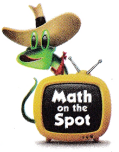It is given that
Maria put trim around a banner that is the shape of a triangle. Each side is 22 inches long. Maria has $$\frac{1}{2}$$ foot of trim left
So,
The total length of the banner = 22 × 3
= 66 inches
Now,
We know that,
1 feet = 12 inches
1 yard = 3 feet
So,
The total length of the banner in feet = $$\frac{66}{12}$$
= 5.5 feet
So,
The total length of the trim when Maria started = 5.5 + 0.5
= 6 feet
So,
The total length of the trim when Maria started in yards = $$\frac{6}{3}$$
= 2 yards
Hence, from the above,
We can conclude that
The total length of the trim when Maria started in yards is: 2 yards

Question 7.
Representations Three lizards ran across a patch of grass. Larry ran 3.6 meters, Lou ran 2,600 millimeters, and Lola ran 310 centimeters. Which statement correctly compares the distances the lizards ran?
(A) Larry ran the farthest.
(B) Lou ran farther than Lola.
(C) Lola ran farther than Larry.
(D) Lola ran the shortest distance.
It is given that
Three lizards ran across a patch of grass. Larry ran 3.6 meters, Lou ran 2,600 millimeters, and Lola ran 310 centimeters
Now,
We know that,
1 meter = 1,000 mm
1 cm = 10 mm
So,
The distance Larry ran = 3.6 × 1,000
= 3,600 mm
The distance Lou ran = 2,600 mm
The distance Lola ran = 310 × 10
= 3,100 mm
Hence, from the above,
We can conclude that
The statement that correctly compares the distances the lizards ran is:Question 8.
Miranda is making lemonade from a powdered mix. She needs to add 3 cups of powdered mix to a large pitcher of water, but she only has a scoop that holds 2 ounces. How many 2-ounce scoops will she use?
(A) 4 scoops
(B) 8 scoops
(C) 10 scoops
(D) 12 scoops
It is given that
Miranda is making lemonade from a powdered mix. She needs to add 3 cups of powdered mix to a large pitcher of water, but she only has a scoop that holds 2 ounces
Now,
We know that,
1 cup = 8 fluid ounces
So,
The number of ounces of powdered mix that needs to add to a large pitcher of water = 3 × 8
= 24 fluid ounces
So,
The number of 2-ounce scoops will Mirands used = $$\frac{24}{2}$$
= 12 2-ounce scoops
Hence, from the above,
We can conclude that
The number of 2-ounce scoops will Mirands used is: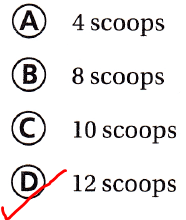Question 9.
Multi-Step Mrs. Gonzalez is adding a border to her bulletin board. She has a strip of green border that is 8 feet long. How many 16-inch pieces can she cut from the strip?
(A) 4 pieces
(B) 6 pieces
(C) 8 pieces
(D) 12 pieces
It is given that
Mrs. Gonzalez is adding a border to her bulletin board. She has a strip of green border that is 8 feet long
Now,
We know that,
1 feet = 12 inches
So,
8 feet = 8 × 12
= 96 inches
Now,
The number of 16-inch pieces can Mrs. Gonzalez cut from the strip = $$\frac{96}{16}$$
= 6  pieces
Hence, from the above,
We can conclude that
The number of 16-inch pieces can Mrs. Gonzalez cut from the strip is:Texas Test Prep

Question 10.
Tony needs 16-inch-long pieces of gold chain to make each of the 3 necklaces. He has a piece of chain that is 4 feet 6 inches long. How much chain will he have left after making the necklaces?
(A) 6 inches
(B) 12 inches
(C) 18 inches
(D) 24 inches
It is given that
Tony needs 16-inch-long pieces of gold chain to make each of the 3 necklaces. He has a piece of chain that is 4 feet 6 inches long.
Now,
We know that,
1 feet = 12 inches
So,
4 feet 6 inches = (4 × 12) + 6
= 54 inches
Now,
The total length of the 3 necklaces = 16 × 3
= 48 inches
So,
The amount of chain will Tony have left after making the necklaces = 54 – 48
= 6 inches
Hence, from the above,
We can conclude that
The amount of chain will Tony have left after making the necklaces is:### Texas Go Math Grade 5 Lesson 13.7 Homework and Practice Answer Key

Question 1.
Jeremy walks through a maze. He begins by walking 25 feet, turning right, and walking 6 yards. Then he walks 32 more feet. He has 13 more yards to walk to complete the maze. What is the length of the maze? Write your answer in yards.
It is given that
Jeremy walks through a maze. He begins by walking 25 feet, turning right, and walking 6 yards. Then he walks 32 more feet. He has 13 more yards to walk to complete the maze
Now,
We know that,
1 feet = $$\frac{1}{3}$$ yard
So,
The total length of the maze = 25 feet + 6 yards + 32 feet + 13 yards
= 57 feet + 19 yards
= $$\frac{57}{3}$$ yards + 19 yards
= 19 yards + 19 yards
= 38 yards
Hence, from the above,
We can conclude that
The total length of the maze is: 38 yards

Question 2.
Randy uses a pedometer to keep track of the distances he walks each day. He discovers that the trip to his mailbox and back is 300 meters. How many kilometers does Randy walk to his mailbox and back if he collects his mail 6 days a week for 4 weeks?
It is given that
Randy uses a pedometer to keep track of the distances he walks each day. He discovers that the trip to his mailbox and back is 300 meters
Now,
We know that,
1 meter = $$\frac{1}{1,000}$$ kilometers
So,
The number of meters does Randy walk to his mailbox and back if he collects his mail 6 days a week for 4 weeks = 300 × 6 × 4 meters
= 300 × 24 meters
= 7,200 meters
So,
The number of kilometers does Randy walk to his mailbox and back if he collects his mail 6 days a week for 4 weeks = 7,200 × $$\frac{1}{1,000}$$
= 7.2 kilometers
Hence, from the above,
We can conclude that
The number of kilometers does Randy walk to his mailbox and back if he collects his mail 6 days a week for 4 weeks is: 7.2 kilometers

Question 3.
Team members are participating in a scavenger hunt. They meet at the starting point and ride their bikes 2 kilometers. They find the first clue that says the second clue is 10 hectometers away. When they reach the second clue, they are told to go back 300 dekameters. Will the team members reach the point where they started after they follow the instructions for the second clue? Explain.
It is given that
Team members are participating in a scavenger hunt. They meet at the starting point and ride their bikes 2 kilometers. They find the first clue that says the second clue is 10 hectometers away. When they reach the second clue, they are told to go back 300 dekameters
Now,
Let us convert our given distance in meters.
So,
1 km = 1,000 meter.
So,
2 km = 2 × 1,000 meters
= 2,000 meters
Now,
1 hectometer = 100 meters
So,
10 hectometers = 10 × 100 meters
= 1,000 meters
Now,
1 Decameter = 10 meters
So,
300 decameters = 300 × 10 meters
= 3,000 meters
Now,
Let us Assume that team members traveled in a straight line.
So,
The distance between the starting point and the 2nd clue = 2,000 + 1,000
= 3,000 meters
Now,
Since the second clue asked the participants to go back 300 decameters,
We will subtract 300 decameters from the total distance covered to reach 2nd clue.
So,
The change in the position = 3,000 – 3,000
= 0
Hence, from the above,
We can conclude that
The team members will reach the point where they started after they follow the instructions for the second clue.

Problem Solving

Question 4.
Mrs. Arnold is serving hamburgers to her family of 6 tonight for dinner. She uses $$\frac{1}{2}$$ pound of ground meat for each hamburger patty. She buys a package of meat that weighs 50 ounces. Will Mrs. Arnold have enough meat? Explain.
It is given that
Mrs. Arnold is serving hamburgers to her family of 6 tonight for dinner. She uses $$\frac{1}{2}$$ pound of ground meat for each hamburger patty. She buys a package of meat that weighs 50 ounces
Now,
The total number of pieces of hamburgers Mrs. Arnold is serving = 6 ÷$$\frac{1}{2}$$
= 6 × 2
= 12 pieces
Now,
We know that,
1 pound = 16 ounces
So,
The total weight of the total number of pieces of hamburgers Mrs. Arnold is serving = 12 × 2 × 16
= 24 × 16
= 384 ounces
So,
384 ounces > 50 ounces
Hence, from the above,
We can conclude that
Mrs. Arnold will have enough meat

Lesson Check

Question 5.
The lengths of three snakes exhibited in the zoo’s reptile house are labeled. The king snake is 65 inches. The eastern diamondback rattlesnake is 2 yards. The ridge-nosed rattlesnake is 4 feet. Which statement correctly compares the lengths of the snakes?
(A) The king snake is the longest snake.
(B) The ridge-nosed rattlesnake is longer than the eastern diamondback rattlesnake.
(C) The eastern diamondback rattlesnake is the longest snake.
(D) The eastern diamondback rattlesnake is shorter than the king snake.
It is given that
The lengths of three snakes exhibited in the zoo’s reptile house are labeled. The king snake is 65 inches. The eastern diamondback rattlesnake is 2 yards. The ridge-nosed rattlesnake is 4 feet
Now,
We know that,
1 feet = 12 inches
1 yard = 36 inches
So,
The length of the King snake = 65 inches
The length of eastern diamondback rattlesnake = 2 ×36 = 72 inches
The length of ridge-nosed rattlesnake = 4 × 12 = 48 inches
Hence, from the above,
We can conclude that
The statement that correctly compares the lengths of the snakes is: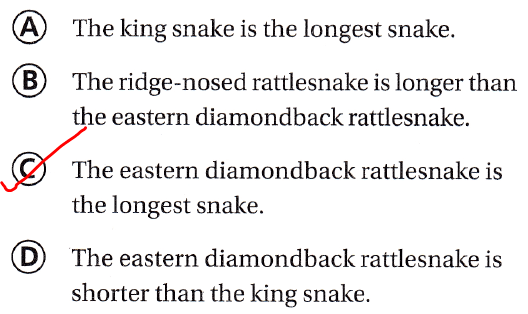Question 6.
Mr. Martinez has 2.5 kilograms of colored sand for his art students to use on their sand art projects. He packages the sand into individual bags with 125 grams in each bag. How many bags of sand can Mr. Martinez make?
(A) 2.5
(B) 16
(C) 20
(D) 200
It is given that
Mr. Martinez has 2.5 kilograms of colored sand for his art students to use on their sand art projects. He packages the sand into individual bags with 125 grams in each bag
Now,
We know that,
1 kg = 1,000 grams
So,
2.5 kilograms = 2.5 × 1,000
= 2,500 grams
So,
The number of bags of sand Mr. Martinez can make = $$\frac{2,500}{125}$$
= 20 bags
Hence, from the above,
We can conclude that
The number of bags of sand Mr. Martinez can make is: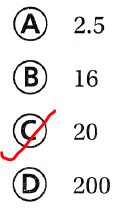Question 7.
A baker needs 6 quarts of milk for a cupcake frosting recipe. He only has an 8-ounce measuring cup to measure the milk into the bowl. How many cups of milk will he use?
(A) 16 cups
(B) 24 cups
(C) 20 cups
(D) 32 cups
It is given that
A baker needs 6 quarts of milk for a cupcake frosting recipe. He only has an 8-ounce measuring cup to measure the milk into the bowl.
Now,
We know that,
1 cup = 8 fluid ounces
1 quart = 32 fluid ounces
So,
6 quarts = 6 × 32
= 192 cups
8 ounces = 1 cup
So,
The number of cups will a baker use = $$\frac{192}{8}$$
= 24 cups
Hence, from the above,
We can conclude that
The number of cups will a baker use is:Question 8.
When Rosa makes lemonade, she uses 500 milliliters of lemon juice for every liter of water. If she made 12 liters of lemonade, how much lemon juice did she use?
(A) 4,000 milliliters
(B) 6,000 milliliters
(C) 8,000 milliliters
(D) 2,000 milliliters
It is given that
When Rosa makes lemonade, she uses 500 milliliters of lemon juice for every liter of water and she made 12 liters of lemonade
Now,
We know that,
1 liter = 1,000 milliliters
So,
12 liters = 12 × 1,000
= 12,000 milliliters
So,
The amount of lemon juice did Rosa used = 12,000 – (12 × 500)
= 12,000 – 6,000
= 6,000 milliliters
Hence, from the above,
We can conclude that
The amount of lemon juice did Rosa used is: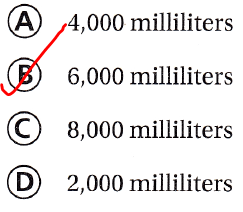Question 9.
Multi-Step A Komodo dragon is 3 meters long. A dwarf gecko is 15 millimeters long. How many dwarf geckos lined up end-to-end would match the length of the Komodo dragon?
(A) 200
(B) 100
(C) 150
(D) 300
It is given that
A Komodo dragon is 3 meters long. A dwarf gecko is 15 millimeters long
Now,
We know that,
1 meter = 1,000 mm
So,
3 meters = 3 × 1,000 mm
= 3,000 mm
So,
The number of dwarf geckos lined up end-to-end would match the length of the Komodo dragon = $$\frac{3,000}{15}$$
= 200
Hence, from the above,
We can conclude that
The number of dwarf geckos lined up end-to-end would match the length of the Komodo dragon is: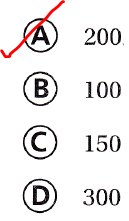Question 10.
Multi-Step Campers use a roll of string that is 250 yards long to make kites. Each kite will have 50 feet of kite line. How many kite lines can they cut from the roll of string?
(A) 5
(B) 50
(C) 150
(D) 15
It is given that
Campers use a roll of string that is 250 yards long to make kites. Each kite will have 50 feet of kite line
Now,
We know that,
1 yard= 3 feet
So,
250 yards = 250 × 3 feet
= 750 feet
So,
The number of kite lines can the Campers cut from the roll of string = $$\frac{750}{50}$$
= 15 kite lines
Hence, from the above,
We can conclude that
The number of kite lines can the Campers cut from the roll of string is: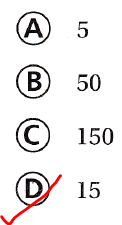Scroll to Top